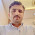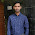## Tuesday, August 21, 2012

### Electrical Machine: Part 1

Electrical Machines Questions and Answers
 A salient pole synchronous motor is running at no load. Its field current is switched off. The motor will
(a) Come to stop.
(b) Continue to run at synchronous speed.
(c) Continue to run at a speed slightly more than the synchronous speed.
(d) Continue to run at a speed slightly less than the synchronous speed.

 The emf induced in the primary of a transformer
(a) Is in phase with the flux.
(b) Lags behind the flux by 90 degree.
(c) Leads the flux by 90 degree.
(d) Is in phase opposition to that of flux.

Ans
wer: C
 The frequency of the rotor current in a 3 phase 50 Hz, 4 pole induction motor at full load speed is about
(a) 50 Hz.
(b) 20 Hz.
(c) 2 Hz.
(d) Zero.

Ans
wer: C
 The two windings of a transformer is
(c) Not linked at all.

Ans
wer : B
  The d.c. series motor should always be started with load because
(a) At no load, it will rotate at dangerously high speed.
(b) It will fail to start.
(c) It will not develop high starting torque.
(d) All are true.

Ans
wer: A
 Out of the following methods of heating the one which is independent of supply
frequency is
(a) Electric arc heating
(b) Induction heating
(c) Electric resistance heating
(d) Dielectric heating

 In a stepper motor the angular displacement
(a) Can be precisely controlled.
(b) It cannot be readily interfaced with micro computer based controller.
(c) The angular displacement cannot be precisely controlled.
(d) It cannot be used for positioning of work tables and tools in NC machines.

Ans
wer: A
 The generation voltage is usually

(a) Between 11 KV and 33 KV.
(b) Between 132 KV and 400 KV.
(c) Between 400 KV and 700 KV.
(d) None of the above.

Ans
wer: A
 When a synchronous motor is running at synchronous speed, the damper winding produces
(a) Damping torque.
(b) Eddy current torque.
(c) Torque aiding the developed torque.
(d) No torque.

Ans
wer: D
 A hysteresis motor
(a) Is not a self-starting motor.
(b) Is a constant speed motor.
(c) Needs dc excitation.
(d) Can not be run in reverse speed.

Ans
wer: B
 If a transformer primary is energized from a square wave voltage source, its output voltage will be
(a) A square wave.
(b) A sine wave.
(c) A triangular wave.
(d) A pulse wave.

Ans: A

 The power factor of a squirrel cage induction motor is
(a) Low at light load only.
(b) Low at heavy load only.
(c) Low at light and heavy load both.
(d) Low at rated load only.

Ans
wer: A
 In a d.c. machine, the armature mmf is
(a) Stationary w.r.t. armature.
(b) Rotating w.r.t. field.
(c) Stationary w.r.t. field.
(d) Rotating w.r.t. brushes.

Ans
wer: C
 In a transformer the voltage regulation will be zero when it operates at
(a) Unity p.f.
(c) Lagging p.f.
(d) Zero p.f. leading.

Ans
wer: B
 The primary winding of a 220/6 V, 50 Hz transformer is energised from 110 V, 60 Hz supply. The secondary output voltage will be
(a) 3.6 V.
(b) 2.5 V.
(c) 3.0 V.
(d) 6.0 V.

Ans
wer: C
 The relative speed between the magnetic fields of stator and rotor under steady state operation is zero for a
(a) DC machine.
(b) 3 phase induction machine.
(c) Synchronous machine.
(d) Single phase induction machine.

Ans
wer: All are correct
 The current from the stator of an alternator is taken out to the external load circuit through
(a) Slip rings.
(b) Commutator segments.
(c) Solid connections.
(d) Carbon brushes.

Ans: C

 A motor which can conveniently be operated at lagging as well as leading power factors is the
(a) Squirrel cage induction motor.
(b) Wound rotor induction motor.
(c) Synchronous motor.
(d) DC shunt motor.

Ans
wer: C
 The most suitable servomotor for low power applications is
(a) A dc series motor.
(b) A dc shunt motor.
(c) An ac two-phase induction motor.
(d) An ac series motor.

Ans
wer: B
 The size of a conductor used in power cables depends on the
(a) Operating voltage.
(b) Power factor.
(c) Current to be carried.
(d) Type of insulation used.

Ans
wer: C
.......................................................................................................

7:24 PM

1.Thanks...

2.please tell me which type of questions are asked in specialist officer paper for techinal in electrical

3.4.Thanks dear for such question and answer but confusion on qestion 11 ans *

5.6.7.Question No.2 the answer is b (i.e; the induced emf in the primary is lagging behind the flux by 90 degrees)